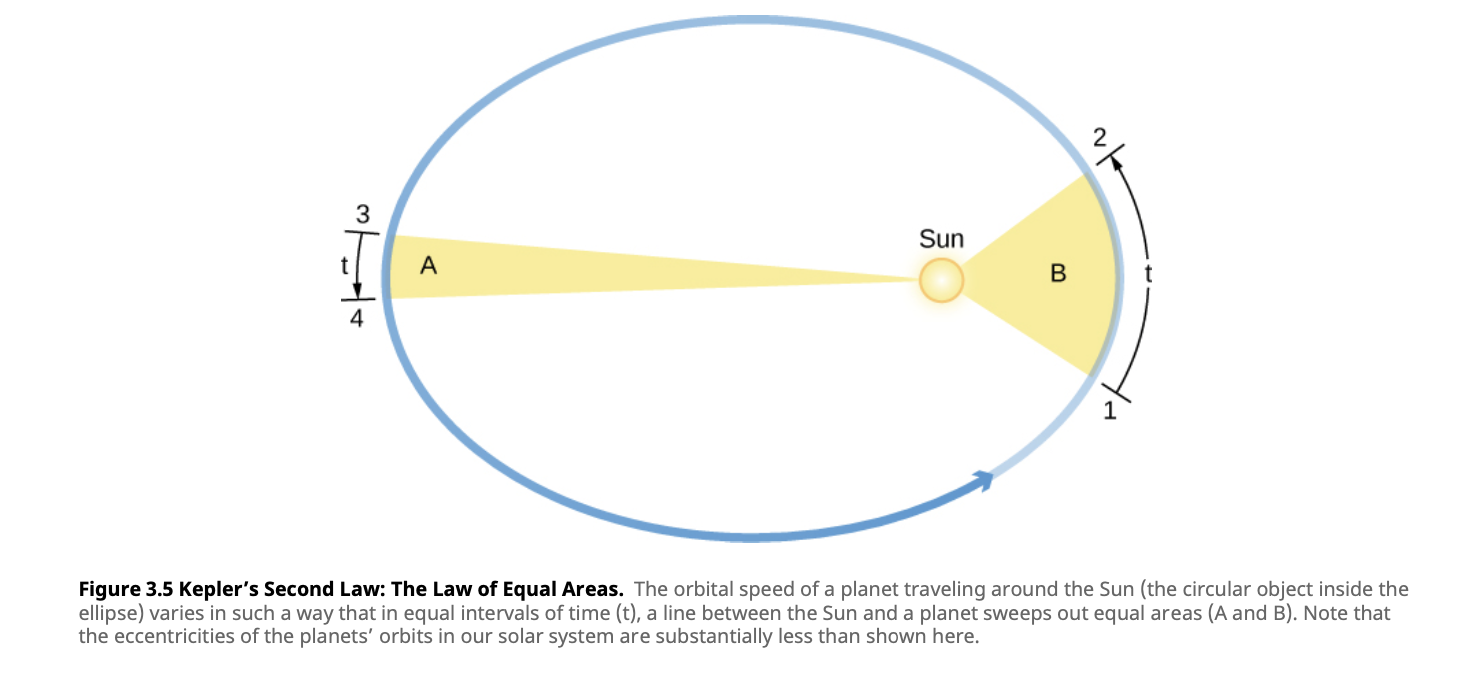# Planetary Motion

## Johannes Kepler's Three Laws of Orbits

1. Each planet orbits the Sun in an ellipse
2. Straight line joining a planet and the Sun sweeps out equal areas in space and equal areas in time
• Eg. in 60 days a planet will move the same amount of area, no matter how close it is to the body it orbits
• The Law of Equal Areas
•• Discovered by observing orbits speeding up as a planet gets closer to the Sun
• Consequence of the conservation of angular momentum
• Figure skater law
3. A planet's orbital period ($P$) squared is proportional to the semi-major axis ($a$) of its orbit cubed
• $P^2 \propto a^3$
• When $P$ is measured in years and $a$ is measured in astronomical units, the two sides are equal
• Applies to all objects orbiting the Sun
• Provides means for calculating objects relative distances from the Sun
• Law of harmonies

### Newton's Addition to the Third Law

• Isaac Newton realized that the Sun's mass and a planets mass come into play with regards to the third law
• The proper formula is $a^3 = (M_1 + M_2) \times P^2$
• $M_1$ is the mass of the Sun
• $M_2$ is the mass of the planet
• Both $M_1$ and $M_2$ are expressed in units of the Sun's mass